Geometry

# Tangent and Secant Lines Warmup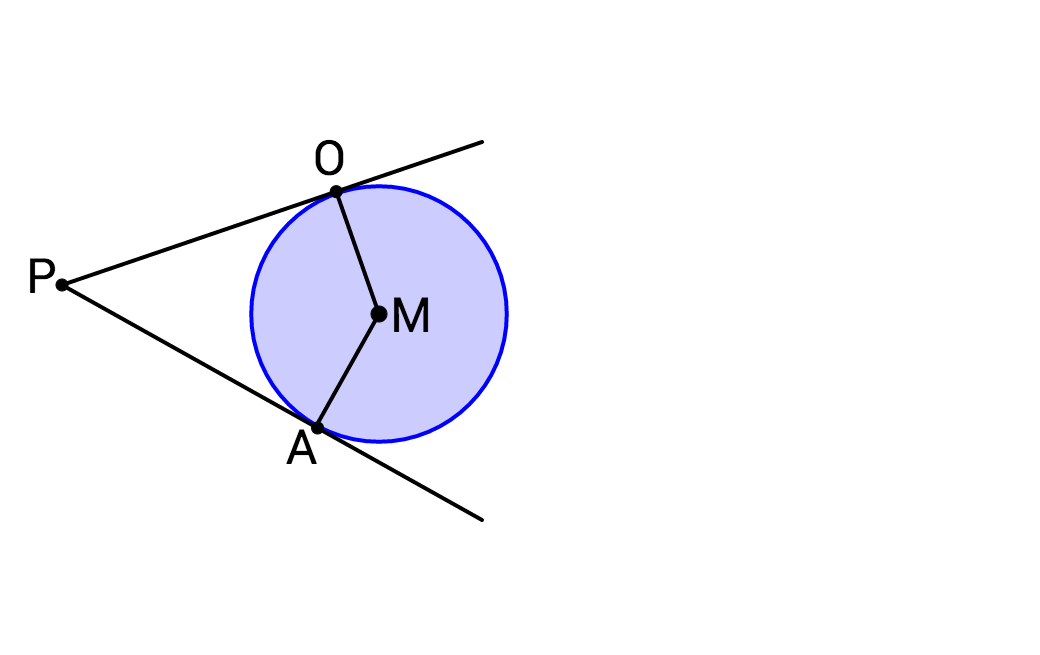Given the two lines tangent to circle $M,$ what is the measure of $\angle POM + \angle PAM ?$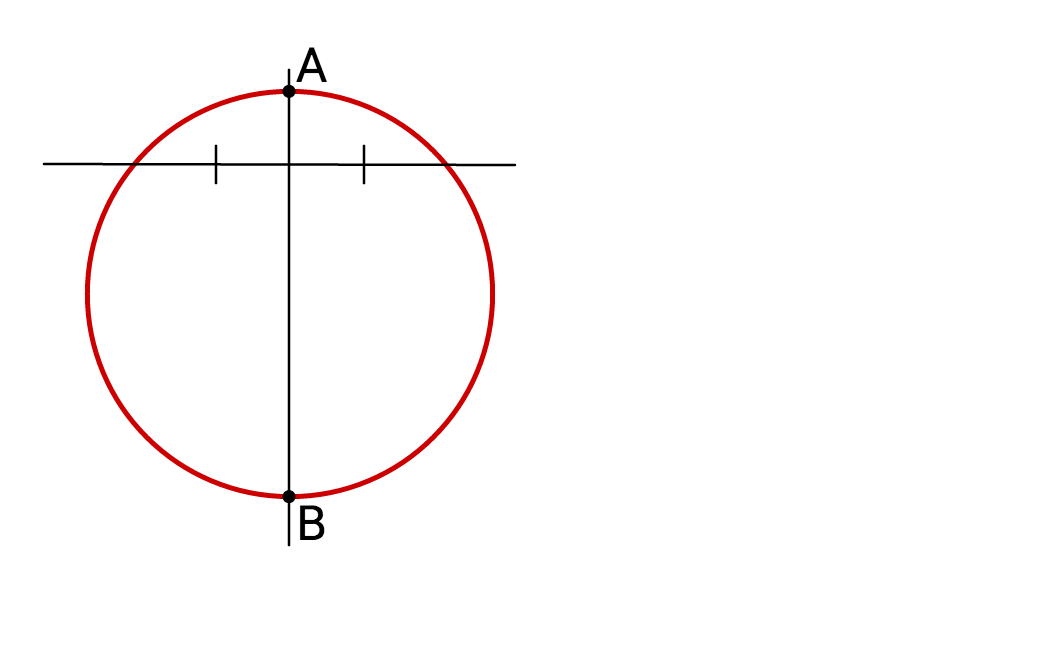The lines shown in the diagram are perpendicular.

True or False: Segment $AB$ must be the diameter of the circle.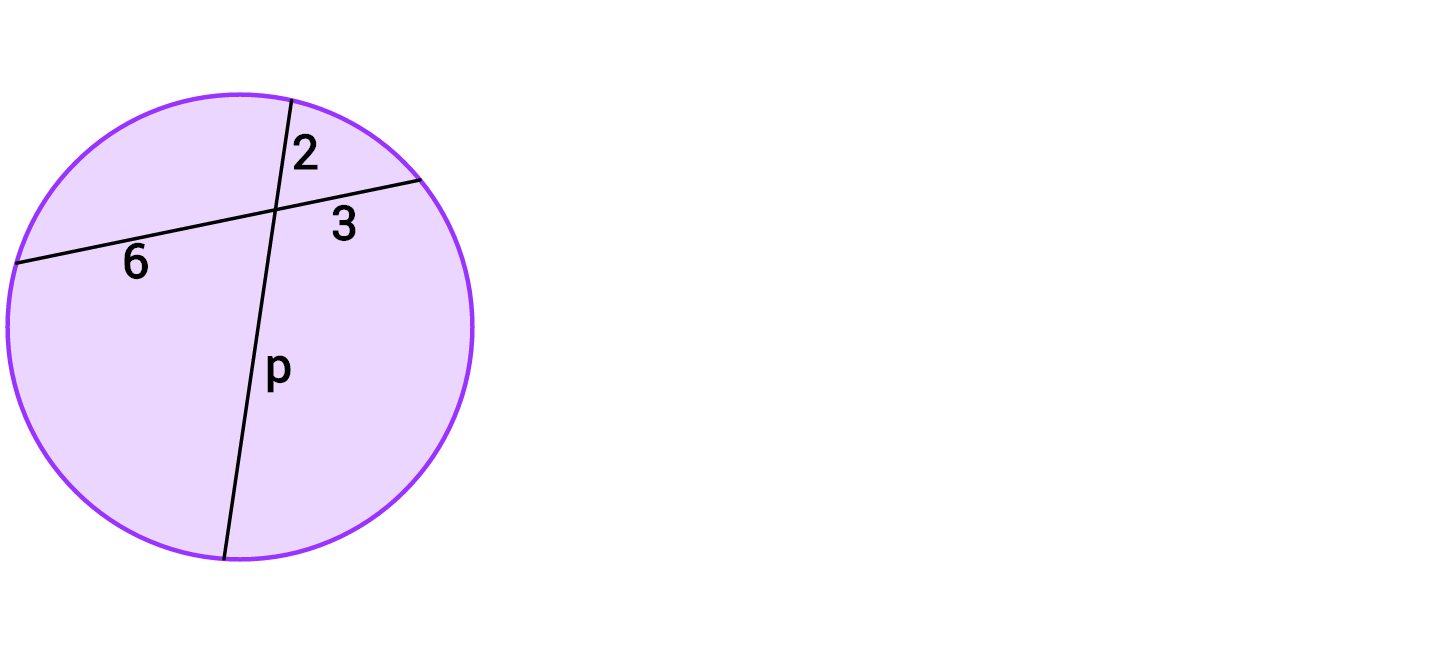What is the value of $p?$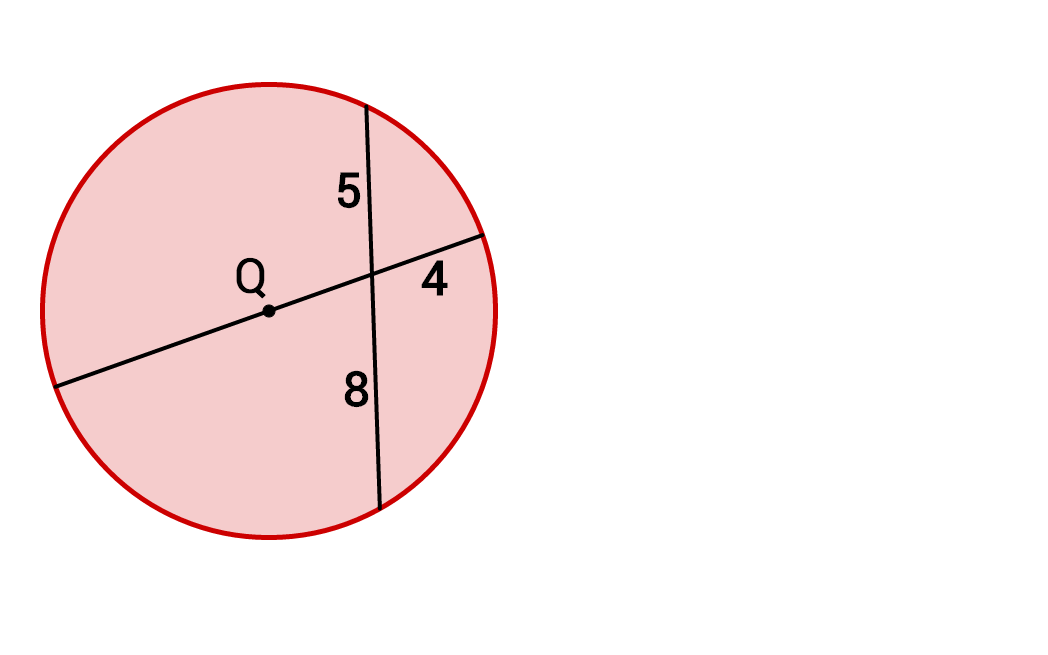What is the radius of circle $Q?$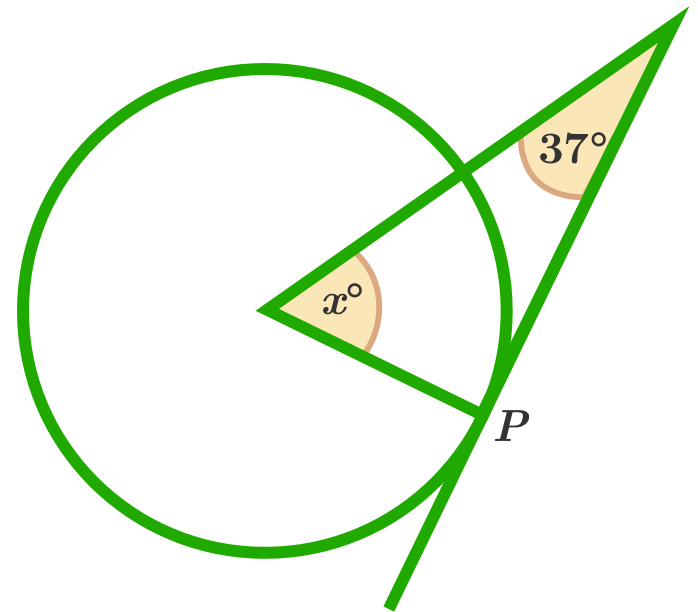If the line is tangent to the circle at point P and the vertex of the angle at $x$ is the center of the circle, what is the value of $x?$

×